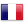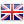﻿ Convert Dynamic Viscosity

# Convert Dynamic Viscosity

Value Unit - Dyne second per square centimetre (dyn·s/cm²) - Pound per foot hour (lb/(ft·h)) - Pound per foot second (lb/(ft·s)) - Pound-force second per square foot (lbf·s/ft²) - Pound-force second per square inch (lbf·s/in²) - Newton second per square metre (N·s/m²) - Gram per centimeter second (g/(cm·s)) - Kilogram per metre second (kg/(m·s)) - Centipoise (cP) - Poise (P) - Pascal second (Pa·s)

Show the entire list of specific conversion for Convert Dynamic ViscosityFREN